# The Number Line

## Greater than or Less than

Putting numbers on a number line makes it easier to decide which one is larger and which one is smaller: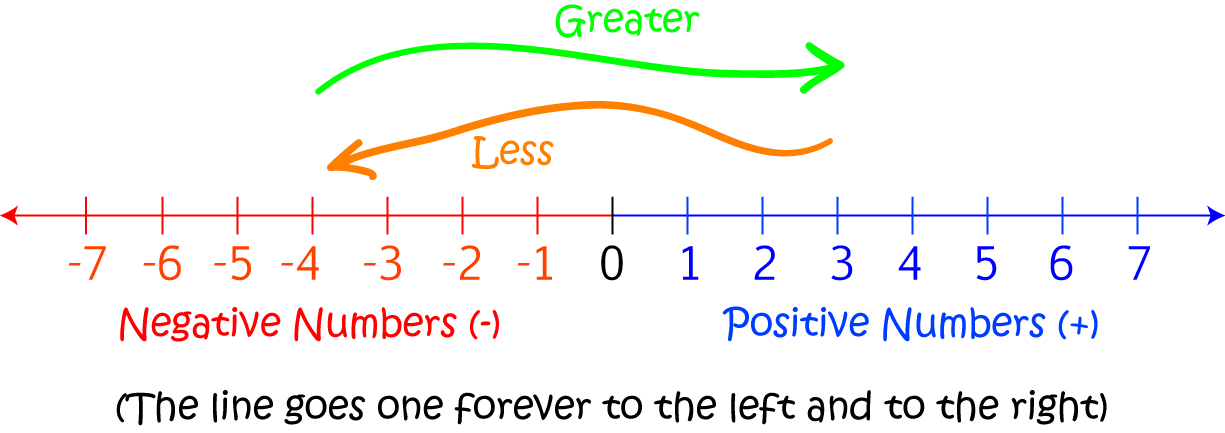Numbers on the left are less than numbers on the right.

Numbers on the right are greater than numbers on the left.

### Examples

#### 1. Less Than$2$ is to the left of $5$, so $2$ is less than $5$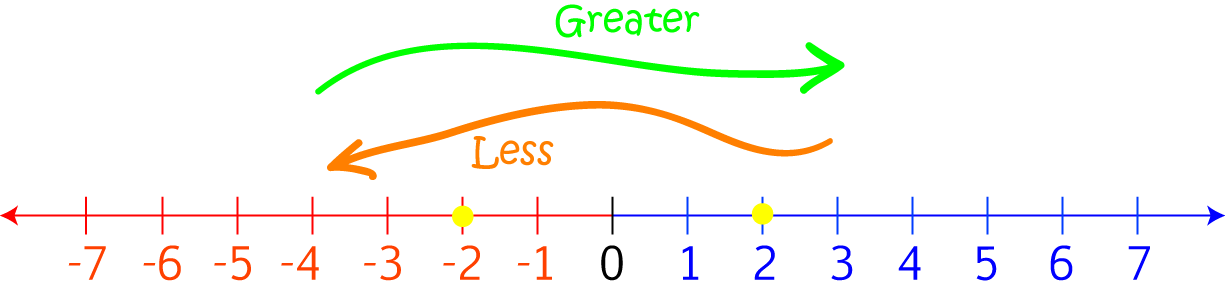$-2$ is to the left of $2$, so $-2$ is less than $2$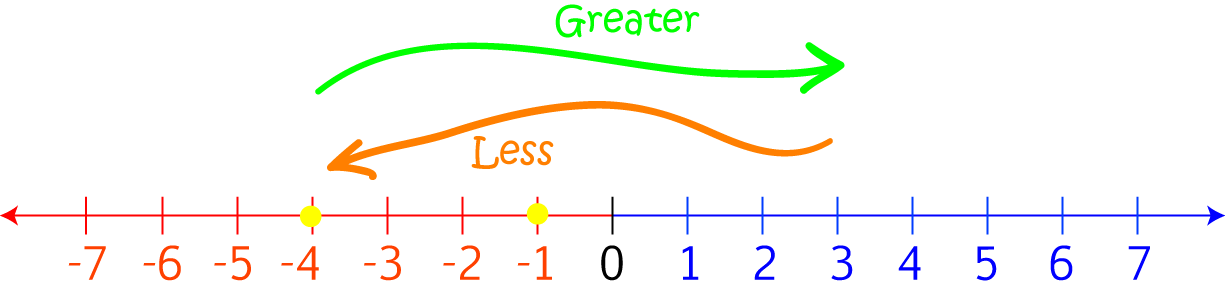$-4$ is to the left of $-1$, so $-4$ is less than $-1$

#### 2. Greater Than$5$ is to the right of $2$, so $5$ is greater than $2$$2$ is to the right of $-2$, so $2$ is greater than $-2$$-1$ is to the right of $-4$, so $-1$ is greater than $-4$

#### 3. Comparing More than 2 Numbers

Jasmin, Sam and Al's mum is trying to get them to eat brussels sprouts. She decides to give them $50 \text{ c}$ for each brussels sprout they eat, and fine them $50 \text{ c}$ for each brussels sprout they give to their dog, Lucy. Lucy loves brussels sprouts! None of them have any money before dinner, and they are all served up the same number of brussels sprouts.

After dinner, Sam owes his mum $3$, Al has $\ 0$ and Jasmin has earned $\2$. Who ate the most brussels sprouts? Who gave the most brussels sprouts to Lucy?

The person who ate the most brussels sprouts will be the one with the most money after dinner. The person who gave the most brussels sprouts to Lucy will be the person who has the least money after dinner.

Place these amounts on the number line to see who has the greatest amount of money and who has the least amount of money.

1. Negative numbers mean that money is owed.
2. Positive numbers mean that someone has money.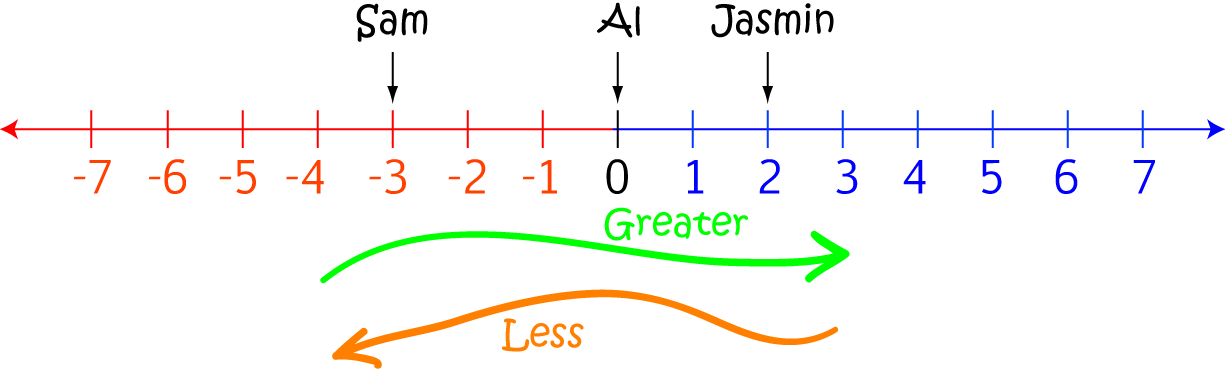Sam's marker is furthest to the left. So, he has the least money, and he must have fed the most brussels sprouts to Lucy.

Jasmin's marker is furthest to the right. So, she has the most money, and she must have eaten the most brussels sprouts.

Al's marker is somewhere in between: he has less money than Jasmin, but more money that Sam. He probably sat at the dinner table going "one for me", "one for Lucy" with his brussels sprouts.

## Using the Number Line

We can also use the number line to help us to do addition and subtraction. When we add, we move to the right along the number line. When we subtract, we move to the left.

### Examples

When we are adding, we move to the right on the number line.

1. Let's use the number line to find out what $2 + 5$ is:We start out at $2$, and then move $5$ places to the right to add on 5. The answer is $7$.

2. Let's use the number line to find out what $-4 + 3$ is: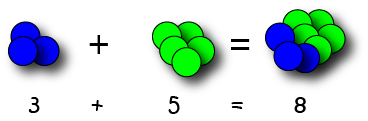We start out at $-4$, and then move $3$ places to the right to add on $3$. The answer is $-1$.

3. Let's use the number line to find out what $-3 + 5$ is:We start out at $-3$, and then move $5$ places to the right to add on 5. The answer is $2$.

#### 2. Subtracting

When we are subtracting, we move to the left on the number line.

1. Let's use the number line to find out what $7 - 6$ is: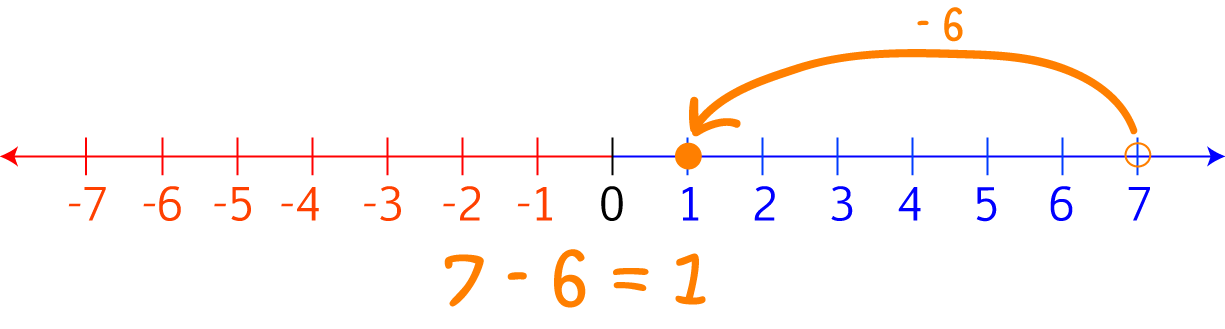We start out at $7$, and then move $6$ places to the left to subtract $6$. The answer is $1$.

2. Let's use the number line to find out what $-1 - 4$ is:We start out at $-1$, and then move $4$ places to the left to subtract $4$. The answer is $-5$.

## Measuring the distance from zero

We can also use the number line to measure how far a number is from zero. This is called the absolute value of the number. To find it, you just count the number of spaces on the number line between the number and zero.For example, "5" is 5 spaces from zero, and so is "-5".

We say that the absolute value of "5" is 5. We also say that the absolute value of "-5" is 5.

Absolute values are always positive numbers because they measure distances.

What do you think would be the absolute value of "4"? How about "-4"?

### Description

This mini book covers the core of Math for Foundation, Grade 1 and Grade 2 mathematics including

1. Numbers
3. Subtraction
4. Division
5. Algebra
6. Geometry
7. Data
8. Estimation
9. Probability/Chance
10. Measurement
11. Time
12. Money
13. and much more

This material is provided free of cost for Parent looking for some tricks for their Prekinder, Kinder, Prep, Year 1 and Year 2 children

### Learning Objectives

These lessons are for kids aged 4-8 with the core objective to expose their brains to concepts of addition, subtraction, division, algebra and much more.

Author: Subject Coach
You must be logged in as Student to ask a Question.